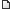It is currently Wed Jan 26, 2022 10:04 pm

Author Message
 Post subject: How good is the MVA method for short circuit calculations?Posted: Fri Sep 06, 2013 11:38 pm

Joined: Mon Oct 08, 2012 2:59 am
Posts: 24
Location: India
I have done a few sample calculations (by hand) of MVA method for short circuit calculations and verified the same with the results with ANSI and IEC 60909 SC calculations in ETAP. I have observed that the MVA method is coming very close to ANSI calculations (error ~ 1% - acceptable error). But the error in IEC calculations is exceeding 1%.
I am interested in IEC method because of local regulations.

The question is - "Is MVA method of short circuit calculations for symmetrical bolted fault current acceptable/good enough for arc flash hazard calculations?"

TopPost subject:Posted: Fri Feb 14, 2014 8:04 am
 Sparks LevelJoined: Wed Jun 10, 2009 5:00 pm
Posts: 267
Location: Toronto
The MVA method is a modification of the Ohmic method where the impedance of a circuit equals the sum of the impedances of components constituting the circuit. Using the admittances, it follows that the reciprocal of the system impedance is the sum of the reciprocals of the admittances of the components. By very definition, the circuit component admittance is the maximum current or KVA at unit voltage which would flow through the circuit or component to a short circuit or fault when supplied from a source of infinite capacity. In practice, the MVA method is used by separating the circuit into components and calculating each component with its own infinite bus.

The disparity between calculation results from ANSI, IEC comprehensive method etc. can be explained by different approximations and assumptions adopted by different methodologies. For example, some techniques ignore equipment X/R ration in the analysis and apply different correction factors to equipment ratings. Disregarding the equipment X/R ratio introduces up to 15% uncertainty in a single step of adding two impedances alone while applying different correction factors does not quantifies and does not corrects the error but only amplifies it.

Both Ohmic and MVA methods are reliable and produce accurate short circuit currents as long as the impedance for equipment (source, transformers, cables etc.) within the electrical system is broken down into X and R components, the components are grouped together and impedance between the source and the fault is calculated using the formula Z = (R^2+X^2)^0.5

_________________
Michael Furtak, C.E.T.